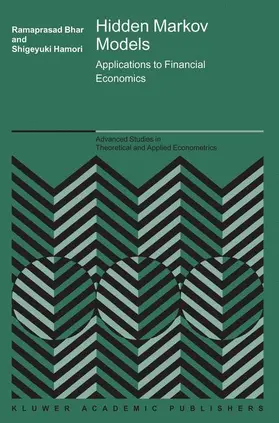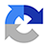# Hamori / BharHidden Markov Models

Applications to Financial Economics

2004, Band: 40, 162 Seiten, Gebunden, HC runder Rücken kaschiert, Format (B × H): 160 mm x 241 mm, Gewicht: 445 g Reihe: Advanced Studies in Theoretical and Applied Economics
ISBN: 978-1-4020-7899-6
Verlag: Springer US

## Hamori / BharHidden Markov Models

Markov chains have increasingly become useful way of capturing stochastic nature of many economic and financial variables. Although the hidden Markov processes have been widely employed for some time in many engineering applications e.g. speech recognition, its effectiveness has now been recognized in areas of social science research as well. The main aim of Hidden Markov Models: Applications to Financial Economics is to make such techniques available to more researchers in financial economics. As such we only cover the necessary theoretical aspects in each chapter while focusing on real life applications using contemporary data mainly from OECD group of countries. The underlying assumption here is that the researchers in financial economics would be familiar with such application although empirical techniques would be more traditional econometrics. Keeping the application level in a more familiar level, we focus on the methodology based on hidden Markov processes. This will, we believe, help the reader to develop more in-depth understanding of the modeling issues thereby benefiting their future research.

Research

## Weitere Infos & Material

List of Figures. List of Tables. Dedication. Acknowledgements. 1: Introduction. 1. Introduction. 2. Markov Chains. 3. Passage Time. 4. Markov Chains and the Term Structure of Interest Rates. 5. State Space Methods and Kalman Filter. 6. Hidden Markov Models and Hidden Markov Experts. 7. HMM Estimation Algorithm. 8. HMM Parameter Estimation. 9. HMM Most Probable State Sequence: Viterbi Algorithm. 10. HMM Illustrative examples. 2: Volatility in Growth Rate of Real GDP. 1. Introduction. 2. Models. 3. Data. 4. Empirical Results. 5. Conclusion. 3: Linkages among G7 Stock Markets. 1. Introduction. 2. Empirical Technique. 3. Data. 4. Empirical Results. 5. Conclusion. 4: Interplay between Industrial Production and Stock Market. 1. Introduction. 2. Markov Switching Heteroscedasticity Model of Output and Equity. 3. Data. 4. Empirical Results. 5. Conclusion. 5: Linking Inflation and Inflation Uncertainty. 1. Introduction. 2. Empirical Technique. 3. Data. 4. Empirical Results. 5. Conclusion. 6: Exploring Permanent and Transitory Components of Stock Return. 1. Introduction. 2. Markov Switching Heteroscedasticity Model of Stock Return. 3. Data. 4. Empirical Results. 5. Conclusion. 7: Exploring the Relationship between Coincident Financial Market Indicators. 1. Introduction. 2. Markov Switching Coincidence Index Model. 3. Data. 4. Empirical Results. 5. Conclusion. References. Index.

## Ihre Fragen, Wünsche oder Anmerkungen

Ihre Nachricht*
Wie möchten Sie kontaktiert werden?
Anrede*
Titel
Vorname*
Nachname*